F Statistics

Suppose that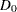is the deviance resulting from fitting a generalized linear model and that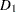is the deviance from fitting a submodel. Then, under appropriate regularity conditions, the asymptotic distribution of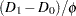is chi-square withdegrees of freedom, whereis the difference in the number of parameters between the two models and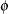is the dispersion parameter. Ifis unknown, andis an estimate ofbased on the deviance or Pearson’s chi-square divided by degrees of freedom, then, under regularity conditions,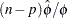has an asymptotic chi-square distribution with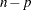degrees of freedom. Here,is the number of observations andis the number of parameters in the model that is used to estimate. Thus, the asymptotic distribution of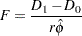is thedistribution withanddegrees of freedom, assuming thatandare approximately independent.

Thisstatistic is computed for the Type 1 analysis, Type 3 analysis, and hypothesis tests specified in CONTRAST statements when the dispersion parameter is estimated by either the deviance or Pearson’s chi-square divided by degrees of freedom, as specified by the DSCALE or PSCALE option in the MODEL statement. In the case of a Type 1 analysis, model 0 is the higher-order model obtained by including one additional effect in model 1. For a Type 3 analysis and hypothesis tests, model 0 is the full specified model and model 1 is the submodel obtained from constraining the Type III contrast or the user-specified contrast to be 0.